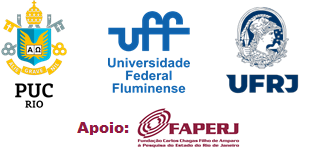Thermodynamical formalism and zero entropy in negative curvature
Felipe Riquelme

Let X be the unit tangent bundle of a complete negatively curved Riemannian manifold and let ( g t ): X → X be its associated geodesic flow. After the work of R. Bowen and D. Ruelle, it is well know that, if X is compact, then any Hölder-continuous potential F : X ? R admits an equilibrium measure. Moreover, there is a fair enough description of some properties of the pressure map t 7→ P ( tF ) , such as its regularity and its asymptotic behaviour. For non-compact situations, the existence of equilibrium measures has been largely studied with success over the last years. However, when we focus the study on properties of the pressure map, there are still many open questions. In this talk we will consider the particular case of geometrically finite manifolds and positive Hölder- continuous potentials with good behaviour near to infinity. We will prove that the pressure map is C 1 and strictly increasing at least on an unbounded interval containing the interval (0 , ∞ ) . Moreover, on those points where the pressure map is locally constant, there are no equilibrium measures. We also prove that the equilibrium measures for tF do not lose mass when t goes to infinity. We compute the entropy of those limit measures, and as an application, we describe the set of entropies of the geodesic flow with respect to ergodic measures. This is a joint work with Anibal Velozo.